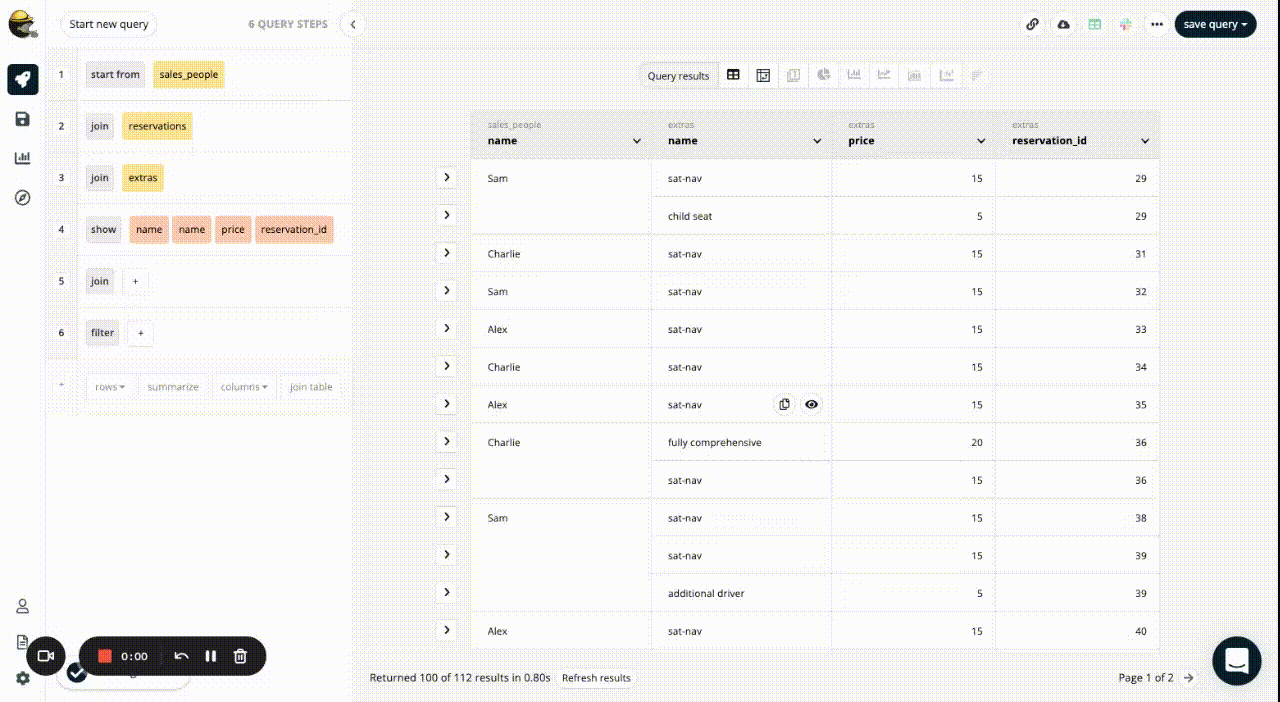# Count unique values

## Count unique values

The count unique values function counts the number of unique values in a specific column.

It's particularly useful for situations where a particular value exists in multiple rows but you only want to count it once.

Example

Let's say we wanted to find the amount of revenue each sales person had generated by selling extras (sat nav, child seats etc). To do this, we'd have to join the sales person table, the reservations table, and the extras table together. It would look like this:

To find the total revenue per sales person, we could just calculate the sum of the price column, and break the results down by the name column.

But, what if we wanted to add some extra context by including a count of the reservations? The problem is, we can't simply count the rows by the reservations ID column because, since we've joined the extras table in and each reservation can be associated with multiple extras, it's possible that there are now multiple rows for each reservation. In fact, if you check the first reservation in the table above (ID 81), you'll see it appears in two rows (since it has two extras associated with it).

The solution is to use the count unique values function. So, starting from the table above, we can 1. calculate the sum of the price column and 2. count the unique values in the reservations ID column, and break both down by the name column. The result would look like this:0 out of 0 found this helpful

Have more questions? Submit a request

<% if (previousArticle || nextArticle) { %>
<% if (previousArticle) { %>
<% if (previousTitle) { %>

#### <%= previousTitle %>

<% } %> <%= previousArticle.title %>
<% } %> <% if (nextArticle) { %>
<% if (nextTitle) { %>

#### <%= nextTitle %>

<% } %> <%= nextArticle.title %>
<% } %>
<% } %>
<% if (items.length > 1) { %>